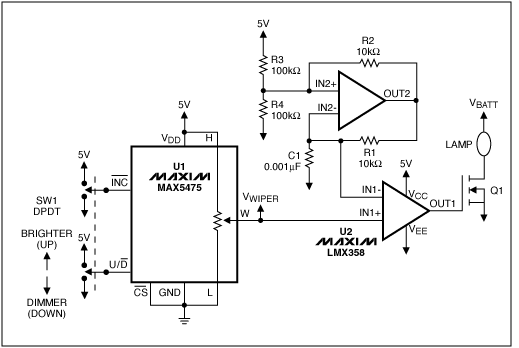# 使用数字分压器实现车灯调光，以节省功耗、提高效率

### 车灯调光方案TDISCHARGE = -R1 × C1 × ln(VIN2+(low)/VIN2+(high)) = 24.1µs(公式1)

TCHARGE = -R1 × C1 × ln{1 - [(VIN2+(high) - VIN2+(low))/(5V - VIN+(low))]} = 32.4µs(公式2)

TPERIOD = TDISCHARGE + TCHARGE = 83.4µs(公式3)

FOSC = 1/TPERIOD = 12kHz